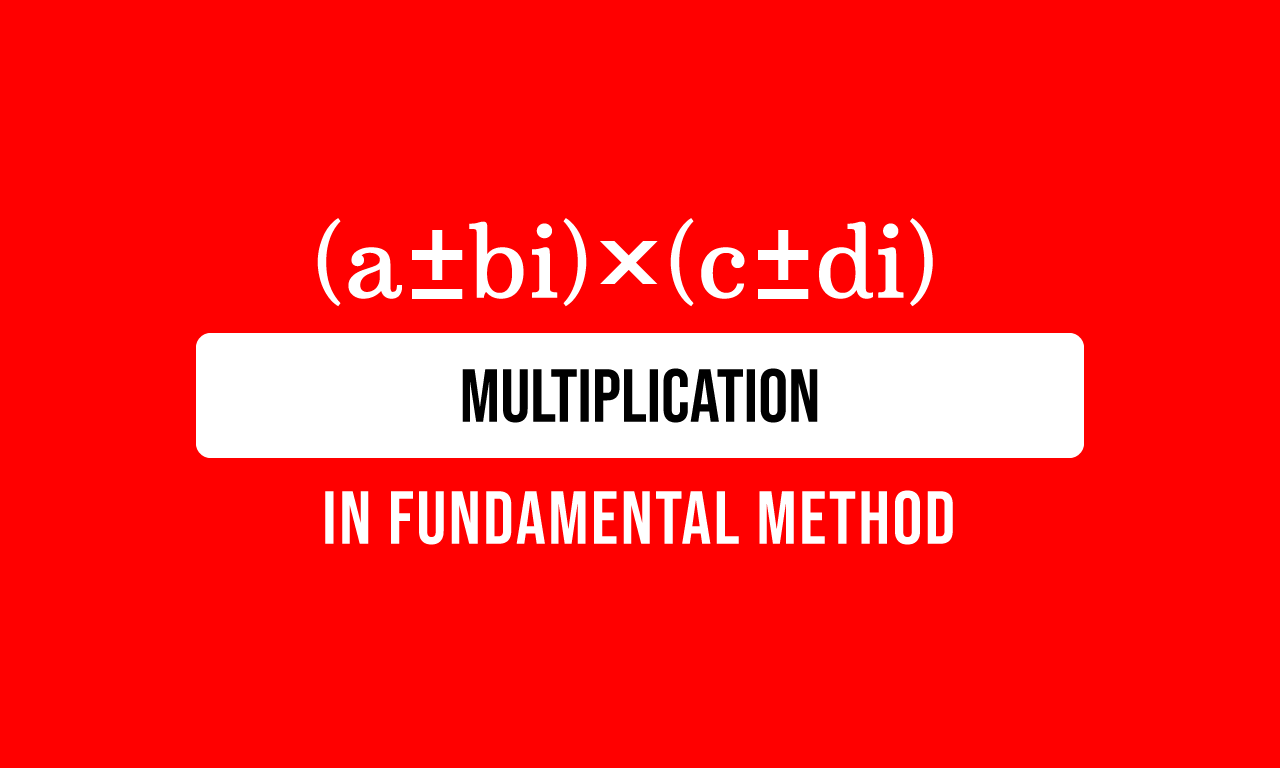# How to Multiply the Complex numbers in fundamental method

The multiplication of the complex numbers can be performed fundamentally by using the following three mathematical concepts. So, you must aware about them before start learning the multiplication of the complex numbers.According to the above fundamental concepts, the complex numbers can be multiplied to find their product in mathematical form. There are two ways for multiplying the complex numbers but the fundamental principle for both methods is same.

The multiplication of the complex numbers is mathematically written in the following form.

$(a+bi) \times (c+di)$

The multiplication of the complex numbers can also be written in product form in mathematics.

$=\,\,\,$ $(a+bi)(c+di)$

## Beginner’s method

It is a simple method, useful for those who gets confusion in multiplying a complex number by another complex number directly.

#### Denote a complex number by a variable

Let’s denote a complex number by a variable. In this case, the complex number $a+bi$ is denoted by $z$ for avoiding the confusion in multiplication.

$=\,\,\,$ $z \times (c+di)$

#### Find the Product by the multiplication

Now, use the distributive property to distribute the coefficient over the addition of the terms.

$=\,\,\,$ $z \times c$ $+$ $z \times di$

Now, replace $z$ by its actual value in the form of a complex number.

$=\,\,\,$ $(a+bi) \times c$ $+$ $(a+bi) \times di$

Now, use the commutative property of multiplication to write the factors in the terms as follows.

$=\,\,\,$ $c \times (a+bi)$ $+$ $di \times (a+bi)$

Now, use the distributive property again to multiply the terms in the complex number by the respective coefficient.

$=\,\,\,$ $c \times a$ $+$ $c \times bi$ $+$ $di \times a$ $+$ $di \times bi$

#### Simplify the mathematical expression

It is time to multiply the factors to find the product of them in each term of above expression.

$=\,\,\,$ $ca$ $+$ $cbi$ $+$ $dia$ $+$ $dbi^2$

Let’s write the factors in each term in our convenient order as per the commutative property of multiplication.

$=\,\,\,$ $ac$ $+$ $bci$ $+$ $adi$ $+$ $bdi^2$

$=\,\,\,$ $ac$ $+$ $bci$ $+$ $adi$ $+$ $bd \times i^2$

Substitute the value of imaginary unit $(i)$ in the above mathematical expression for simplifying it further.

$=\,\,\,$ $ac$ $+$ $bci$ $+$ $adi$ $+$ $bd \times (-1)$

$=\,\,\,$ $ac$ $+$ $bci$ $+$ $adi$ $-$ $bd$

$=\,\,\,$ $ac-bd$ $+$ $bci$ $+$ $adi$

Look at the third and fourth terms in the above mathematical expression. The imaginary unit $i$ is a common factor in both terms. So, it can be taken out common from them.

$=\,\,\,$ $(ac-bd)$ $+$ $i(bc+ad)$

$=\,\,\,$ $(ac-bd)$ $+$ $(bc+ad)i$

$\therefore\,\,\,$ $(a+bi) \times (c+di)$ $\,=\,$ $(ac-bd)$ $+$ $(bc+ad)i$

The mathematical equation can also be written in product form as follows.

$\therefore\,\,\,$ $(a+bi)(c+di)$ $\,=\,$ $(ac-bd)$ $+$ $(bc+ad)i$

It is an advanced method, which can be easily understood by those who have good knowledge on mathematical concepts.

#### Multiply the terms by a complex number

The complex number $a+ib$ is a coefficient of the complex number $c+id$. So, multiply the terms in the complex number $c+id$ by the coefficient as per the distributive property of the multiplication.

$=\,\,\,$ $(a+ib) \times c$ $+$ $(a+ib) \times id$

The factors in each term in the mathematical expression can be written as follows as per the commutative property of the multiplication.

$=\,\,\,$ $c \times (a+ib)$ $+$ $id \times (a+ib)$

#### Multiply the terms by respective coefficient

Now, use the distributive property of multiplication one more time to multiply the terms by its coefficient in every term of the mathematical expression.

$=\,\,\,$ $c \times a$ $+$ $c \times ib$ $+$ $id \times a$ $+$ $id \times ib$

#### Simplify the mathematical expression

Now, find the product of each term in the above mathematical expression.

$=\,\,\,$ $ca$ $+$ $cib$ $+$ $ida$ $+$ $i^2db$

The value of imaginary unit $i$ can be substituted in the fourth term of the mathematical expression for simplifying it further.

$=\,\,\,$ $ca$ $+$ $cib$ $+$ $ida$ $+$ $(-1)db$

$=\,\,\,$ $ca$ $+$ $cib$ $+$ $ida$ $-$ $db$

The factors in each term can be written in an order for our convenience as per the commutative rules.

$=\,\,\,$ $ac$ $+$ $ibc$ $+$ $iad$ $-$ $bd$

$=\,\,\,$ $ac-bd$ $+$ $ibc+iad$

$=\,\,\,$ $ac-bd$ $+$ $iad+ibc$

The imaginary unit is a common factor in both third and fourth terms of the mathematical expression, and it can be taken common from them by taking the common factor out from the terms.

$=\,\,\,$ $ac-bd$ $+$ $i(ad+bc)$

$\therefore\,\,\,$ $(a+bi) \times (c+di)$ $\,=\,$ $(ac-bd)$ $+$ $i(bc+ad)$

Similarly, the multiplication of the complex numbers can also be written in product form as follows.

$\therefore\,\,\,$ $(a+bi)(c+di)$ $\,=\,$ $(ac-bd)$ $+$ $i(bc+ad)$

Latest Math Topics
Jun 26, 2023
Jun 23, 2023

###### Math Questions

The math problems with solutions to learn how to solve a problem.

Learn solutions

Practice now

###### Math Videos

The math videos tutorials with visual graphics to learn every concept.

Watch now

###### Subscribe us

Get the latest math updates from the Math Doubts by subscribing us.